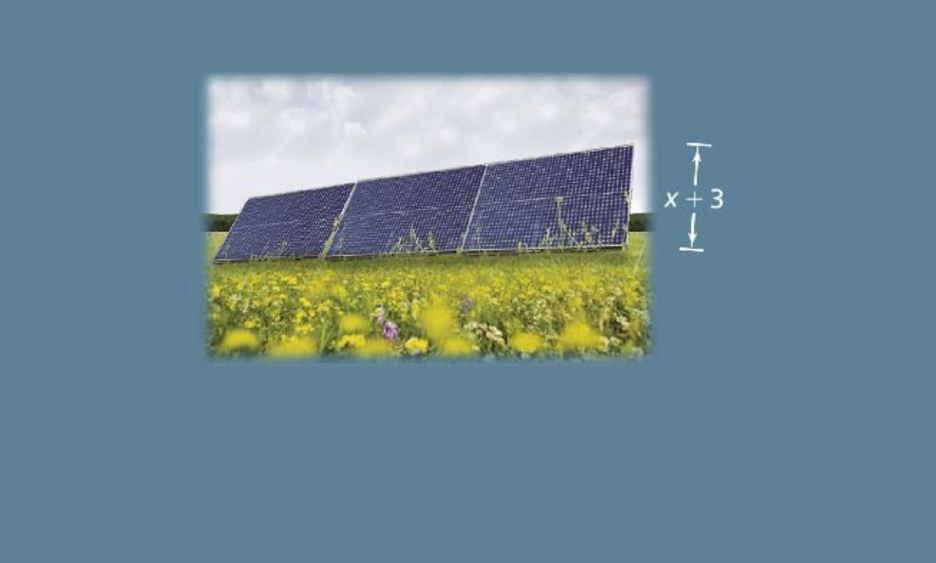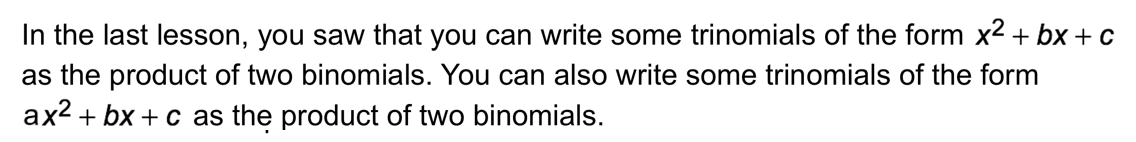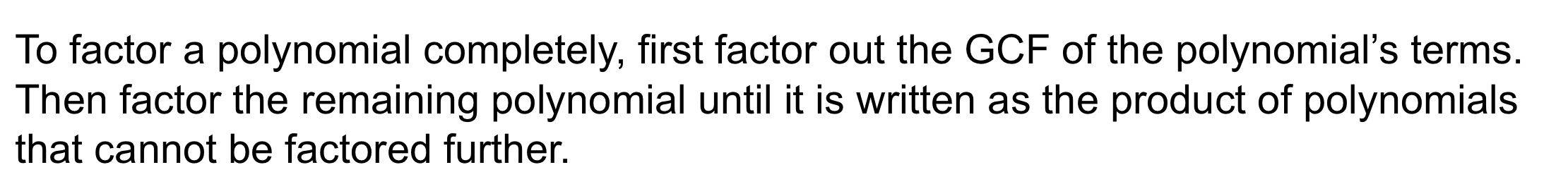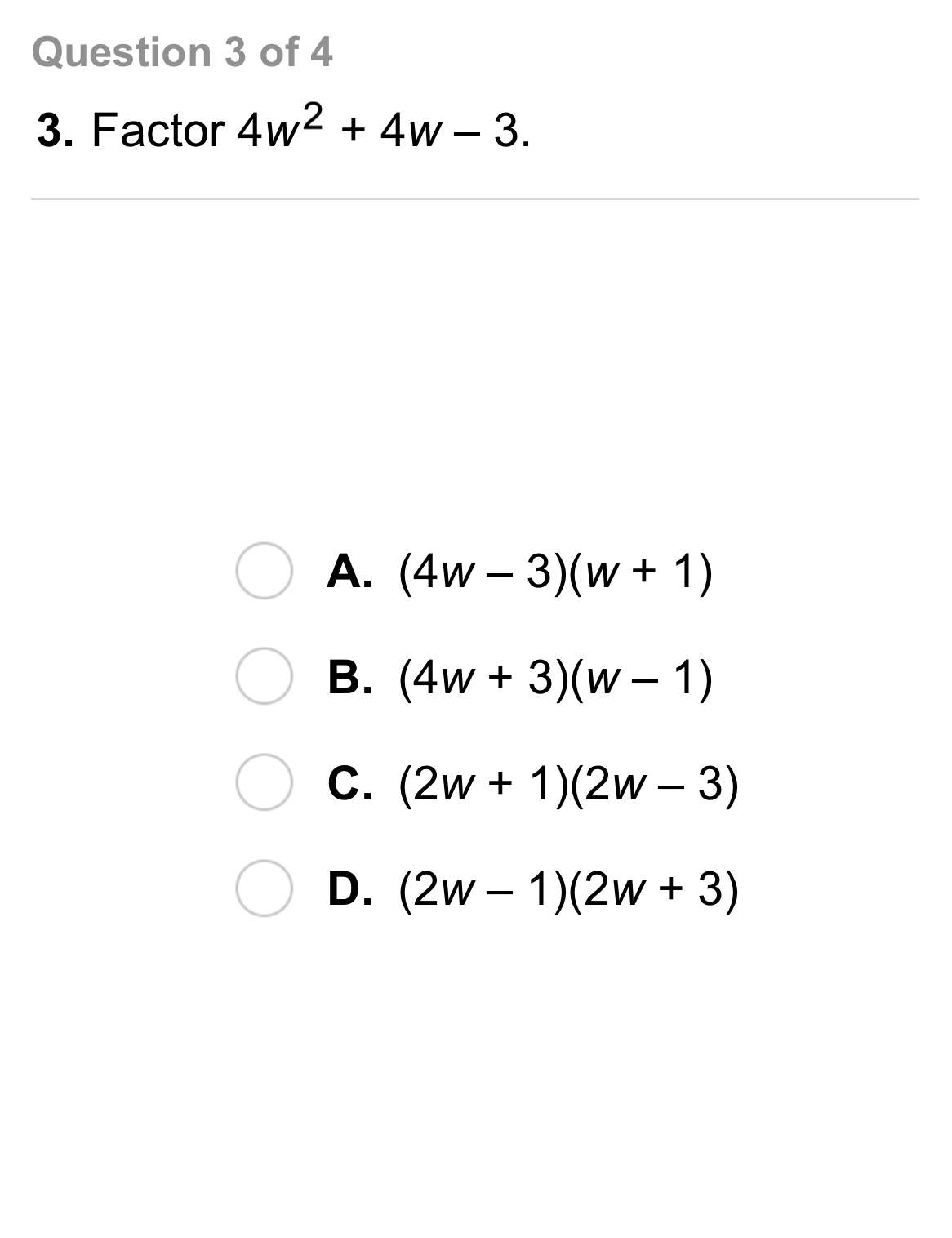Algebra 1 8-6 Complete Lesson: Factoring ax² + bx+ c
starstarstarstarstarstarstarstarstarstar
4 (1 rating)
by Matthew Richardson
| 23 Questions
Note from the author:
A complete formative lesson with embedded slideshow, mini lecture screencasts, checks for understanding, practice items, mixed review, and reflection. I create these assignments to supplement each lesson of Pearson's Common Core Edition Algebra 1, Algebra 2, and Geometry courses. See also mathquest.net and twitter.com/mathquestEDU.The outlined content above was added from outside of Formative.1
1
10 pts
Solve It! An array of three rectangular solar panels has the area below:
The height of the array is x + 3. What is the length of the array?2
10 pts
Problem 1 Got It? Drag two binomial factors to the trinomial to represent its factored form.
• (3x + 1)
• (3x + 5)
• (2x + 1)
• (3x + 2)
• 6x² + 13x + 5
3
10 pts
Problem 1 Got It? Reasoning: Consider the trinomial below.
Suppose ac is positive and b is negative. What do you know about the factors of ac?
One of the factors of ac is positive and the other is negative.
The factors of ac are both positive.
The factors of ac are both negative.4
4
10 pts
Problem 2 Got It?
A
B
C
D5
5
10 pts
Problem 3 Got It?
A
B
C
D6
6
10 pts
Problem 4 Got It?
A
B
C
D7
7
10 pts
A
B
C
D8
8
10 pts
A
B
C
D9
9
10 pts
A
B
C
D10
10
10 pts
A
B
C
D11
10 pts
Reasoning: Explain why you cannot factor the trinomial.

12
10 pts
Reasoning: To factor the trinomial 8x² + bx + 3, a student correctly rewrites the trinomial as 8x² + px + qx + 3.

What is the value of pq? Enter only a number.14
10 pts
Review Lesson 8-5: Identify the factored form of each expression. Check your answer.

• (t + 4)(t + 11)
• (t - 5)(t - 12)
• (t - 4)(t + 7)
15
10 pts
Review Lesson 2-7: Match each proportion with its solution.

• 21
• 12.5
• 12
16
5 pts
Review Lesson 8-4: Simplify the product.

17
5 pts
Review Lesson 8-4: Simplify the product.

18
5 pts
Review Lesson 8-4: Simplify the product.

19
5 pts
Review Lesson 8-4: Simplify the product.20
10 pts
Vocabulary Review: Complete each sentence with the correct form of the word factor.
• factoring
• factor
• factored
• The monomials 12x, 3x², and 6x³ have a common __?__ of 3x.
• When __?__ a trinomial, begin by looking for a factor common to all three terms.
• A student __?__ the expression 12x³ - 6x² into 6x²(2x - 1).
21
10 pts
Use Your Vocabulary: You can rewrite the expression below in order to factor it.
Which rewritten expression below is NOT equivalent to the original expression?

22
100 pts
Notes: Take a clear picture or screenshot of your Cornell notes for this lesson. Upload it to the canvas. Zoom and pan as needed.

For a refresher on the Cornell note-taking system, click here.
23
10 pts
Reflection: Math Success
Add to my formatives list

Formative uses cookies to allow us to better understand how the site is used. By continuing to use this site, you consent to the Terms of Service and Privacy Policy.